• 求取的角度是负值，我在图中画了一个垂直的形状，得出的结果是0 ，原因大家自己去理解。 废话不说，直接上代码： #include #include #include #include #include #include using namespace std; using ...
求取的角度是负值，我在图中画了一个垂直的形状，得出的结果是0 ，原因大家自己去理解。
废话不多说，直接上代码：
#include <iostream>
#include <vector>
#include <opencv2/opencv.hpp>
#include <opencv2/core/core.hpp>
#include <opencv2/imgproc/imgproc.hpp>
#include <opencv2/highgui/highgui.hpp>

using namespace std;
using namespace cv;

int main()
{
RNG rng(12345);
if (!pSrcImage.data)
return 0;
imshow("Image", pSrcImage);

// 转为2值图
threshold(pSrcImage, pSrcImage, 200, 255, cv::THRESH_BINARY_INV);

imwrite("binary.jpg", pSrcImage);

vector<std::vector<cv::Point>> contours;
Mat canny_output;
Canny(pSrcImage, canny_output, 80, 255, 3);
imshow("Canny Image",canny_output);

vector<Vec4i> hierarchy;
findContours(canny_output, contours, hierarchy, CV_RETR_EXTERNAL, CV_CHAIN_APPROX_SIMPLE, Point(0, 0));

/// 对每个找到的轮廓创建可倾斜的边界框和椭圆
vector<RotatedRect> minRect(contours.size());
for (unsigned int i = 0; i < contours.size(); i++)
{
minRect[i] = minAreaRect(Mat(contours[i]));
}

/// 绘出轮廓及其可倾斜的边界框
CvBox2D  End_Rage2D;
CvPoint2D32f rectpoint;
Point2f rect_points;

Mat drawing = Mat::zeros(canny_output.size(), CV_8UC3);
for (unsigned int i = 0; i< contours.size(); i++)
{

End_Rage2D = minAreaRect(Mat(contours[i]));
Scalar color = Scalar(rng.uniform(0, 255), rng.uniform(0, 255), rng.uniform(0, 255));
// contour
drawContours(drawing, contours, i, color, 1, 8, vector<Vec4i>(), 0, Point());
// rotated rectangle
Point2f rect_points; minRect[i].points(rect_points);
for (unsigned int j = 0; j < 4; j++)
{
line(drawing, rect_points[j], rect_points[(j + 1) % 4], color, 1, 8);
cout << "坐标点："<<rect_points[j] << "........" << rect_points[(j + 1) % 4] << endl;
}

int a = 0, b = 0, k = 0;
a = (int)sqrt((pow((rect_points.x - rect_points.x), 2) + pow((rect_points.y - rect_points.y), 2)));
b = (int)sqrt((pow((rect_points.x - rect_points.x), 2) + pow((rect_points.y - rect_points.y), 2)));

if (a<b)
{
k = a;
a = b;
b = k;
}
printf("最小外接矩形的长为：%d，宽为：%d。\n\n", a, b);
cout << " angle:\n" << 90+(float)End_Rage2D.angle << std::endl;      //旋转角度
}

namedWindow("Contours1", CV_WINDOW_AUTOSIZE);
imshow("Contours1", drawing);

waitKey(0);
return 0;

}实验图：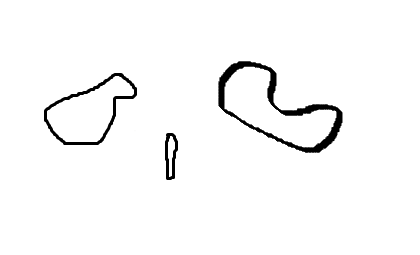实验结果：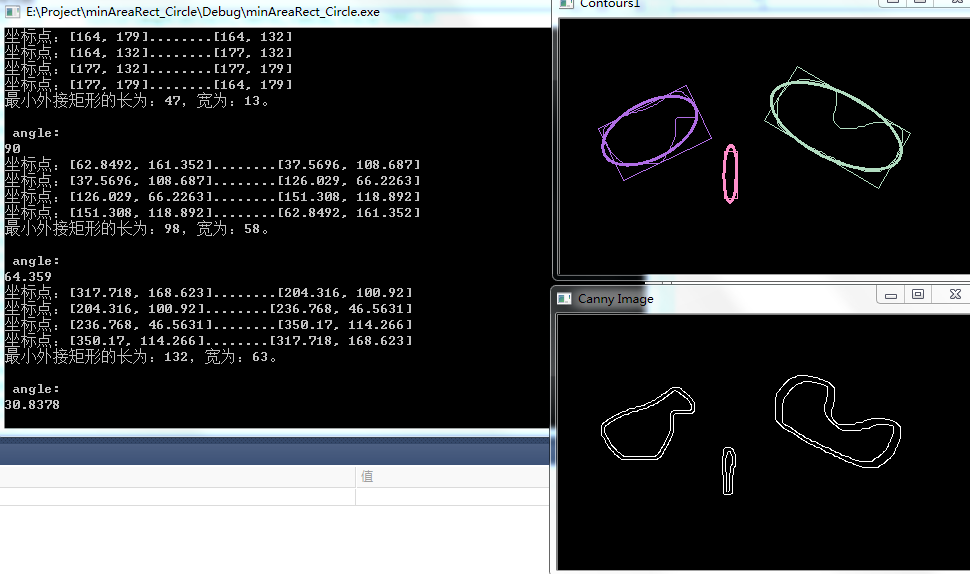展开全文最小外接矩形 opencv
• private Vector3 oldVector3; private Vector3 newVector3; private Quaternion oldQuaternion; private Vector3 newRotatinForward; 初始化 oldVector3 = transform.position; oldQuaternion = ...newRot...


private Vector3 oldVector3;
private Vector3 newVector3;
private Quaternion oldQuaternion;
private Vector3 newRotatinForward;



初始化

oldVector3 = transform.position;
oldQuaternion = transform.rotation

计算

newVector3 = this.transform.position;
newRotatinForward = this.transform.forward;

float lenth = Vector3.Distance(oldVector3, newVector3);
Vector3 velocity = Quaternion.Inverse(oldQuaternion) * newRotatinForward;
float angle = Mathf.Atan2(velocity.x, velocity.z) * 180.0f / 3.14159f;
Debug.Log(angle);


展开全文• ## OpenCV轮廓检测，计算物体旋转角度

万次阅读 热门讨论 2015-02-27 00:54:37
//代入cvMinAreaRect2这个函数得到最小包围矩形 这里已得出被测物体角度，宽度,高度，和中点坐标点存放在CvBox2D类型的结构体中， //主要工作基本结束。 for(int i = 0;i;i++) { //CvArr* s=(CvArr*)&...


效果还是有点问题的，希望大家共同探讨一下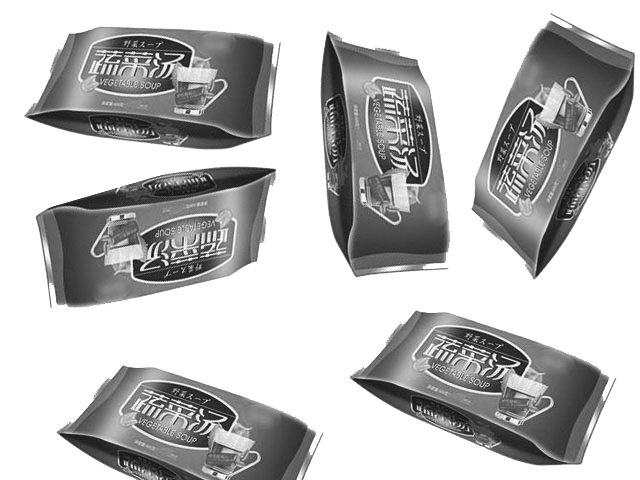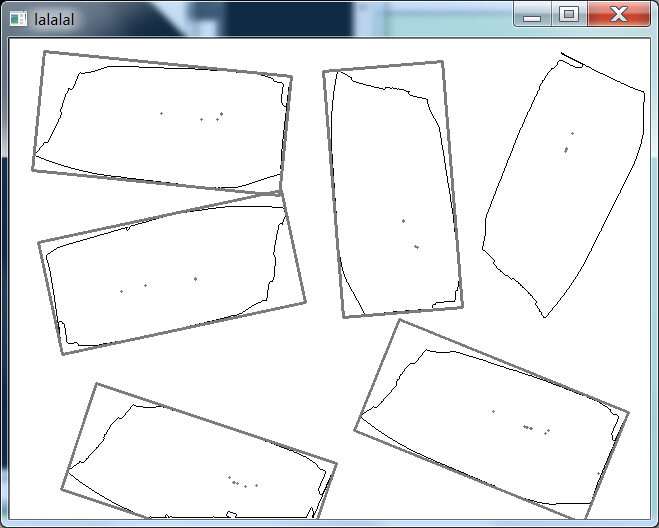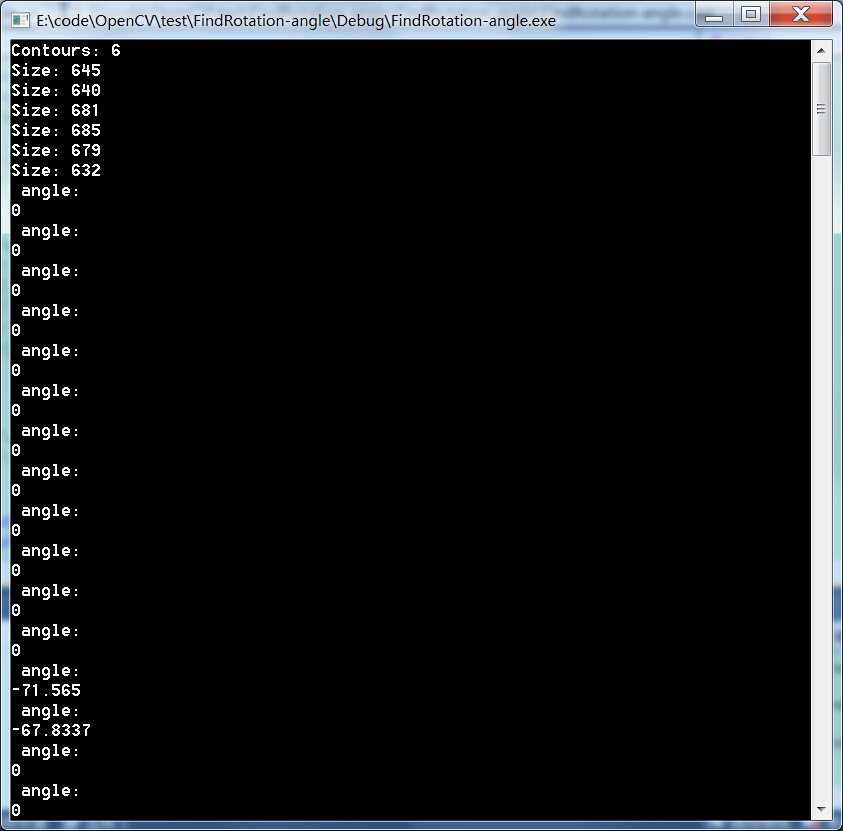// FindRotation-angle.cpp : 定义控制台应用程序的入口点。
//

// findContours.cpp : 定义控制台应用程序的入口点。
//

#include "stdafx.h"

#include <iostream>
#include <vector>
#include <opencv2/opencv.hpp>
#include <opencv2/core/core.hpp>
#include <opencv2/imgproc/imgproc.hpp>
#include <opencv2/highgui/highgui.hpp>

#pragma comment(lib,"opencv_core2410d.lib")
#pragma comment(lib,"opencv_highgui2410d.lib")
#pragma comment(lib,"opencv_imgproc2410d.lib")

#define PI 3.1415926

using namespace std;
using namespace cv;

int hough_line(Mat src)
{
//【1】载入原始图和Mat变量定义
Mat midImage,dstImage;//临时变量和目标图的定义

//【2】进行边缘检测和转化为灰度图
Canny(srcImage, midImage, 50, 200, 3);//进行一此canny边缘检测
cvtColor(midImage,dstImage, CV_GRAY2BGR);//转化边缘检测后的图为灰度图

//【3】进行霍夫线变换
vector<Vec4i> lines;//定义一个矢量结构lines用于存放得到的线段矢量集合
HoughLinesP(midImage, lines, 1, CV_PI/180, 80, 50, 10 );

//【4】依次在图中绘制出每条线段
for( size_t i = 0; i < lines.size(); i++ )
{
Vec4i l = lines[i];
line( dstImage, Point(l, l), Point(l, l), Scalar(186,88,255), 1, CV_AA);
}

//【5】显示原始图
imshow("【原始图】", srcImage);

//【6】边缘检测后的图
imshow("【边缘检测后的图】", midImage);

//【7】显示效果图
imshow("【效果图】", dstImage);

//waitKey(0);

return 0;
}

int main()
{

char *image_name = "test.jpg";
if (!image.data)
return 0;

cv::namedWindow("Binary Image");
cv::imshow("Binary Image",image);

// 从文件中加载原图

// 转为2值图

cvThreshold(pSrcImage,pSrcImage,200,255,cv::THRESH_BINARY_INV);

image = cv::Mat(pSrcImage,true);

cv::imwrite("binary.jpg",image);

// Get the contours of the connected components
std::vector<std::vector<cv::Point>> contours;
cv::findContours(image,
contours, // a vector of contours
CV_RETR_EXTERNAL, // retrieve the external contours
CV_CHAIN_APPROX_NONE); // retrieve all pixels of each contours

// Print contours' length
std::cout << "Contours: " << contours.size() << std::endl;
std::vector<std::vector<cv::Point>>::const_iterator itContours= contours.begin();
for ( ; itContours!=contours.end(); ++itContours)
{

std::cout << "Size: " << itContours->size() << std::endl;
}

// draw black contours on white image
cv::Mat result(image.size(),CV_8U,cv::Scalar(255));
cv::drawContours(result,contours,
-1, // draw all contours
cv::Scalar(0), // in black
2); // with a thickness of 2

cv::namedWindow("Contours");
cv::imshow("Contours",result);

// Eliminate too short or too long contours
int cmin= 100;  // minimum contour length
int cmax= 1000; // maximum contour length
std::vector<std::vector<cv::Point>>::const_iterator itc= contours.begin();
while (itc!=contours.end()) {

if (itc->size() < cmin || itc->size() > cmax)
itc= contours.erase(itc);
else
++itc;
}

// draw contours on the original image
cv::drawContours(original,contours,
-1, // draw all contours
cv::Scalar(255,255,0), // in white
2); // with a thickness of 2

cv::namedWindow("Contours on original");
cv::imshow("Contours on original",original);

// Let's now draw black contours on white image
result.setTo(cv::Scalar(255));
cv::drawContours(result,contours,
-1, // draw all contours
cv::Scalar(0), // in black
1); // with a thickness of 1

//imshow("lll",result);
//waitKey(0);

// testing the bounding box
//
//霍夫变换进行直线检测，此处使用的是probabilistic Hough transform（cv::HoughLinesP）而不是standard Hough transform（cv::HoughLines）

cv::Mat result_line(image.size(),CV_8U,cv::Scalar(255));
result_line = result.clone();

hough_line(result_line);

//Mat tempimage;

//【2】进行边缘检测和转化为灰度图
//Canny(result_line, tempimage, 50, 200, 3);//进行一此canny边缘检测
//imshow("canny",tempimage);
//waitKey(0);

//cvtColor(tempimage,result_line, CV_GRAY2BGR);//转化边缘检测后的图为灰度图
vector<Vec4i> lines;

cv::HoughLinesP(result_line,lines,1,CV_PI/180,80,50,10);

for(int i = 0; i < lines.size(); i++)
{
line(result_line,cv::Point(lines[i],lines[i]),cv::Point(lines[i],lines[i]),Scalar(0,0,0),2,8,0);
}
cv::namedWindow("line");
cv::imshow("line",result_line);
//waitKey(0);

/
//

//std::vector<std::vector<cv::Point>>::const_iterator itc_rec= contours.begin();
//while (itc_rec!=contours.end())
//{
//	cv::Rect r0= cv::boundingRect(cv::Mat(*(itc_rec)));
//	cv::rectangle(result,r0,cv::Scalar(0),2);
//		++itc_rec;
//}

//cv::namedWindow("Some Shape descriptors");
//cv::imshow("Some Shape descriptors",result);

CvBox2D     End_Rage2D;
CvPoint2D32f rectpoint;
CvMemStorage *storage = cvCreateMemStorage(0);  //开辟内存空间

CvSeq*      contour = NULL;     //CvSeq类型 存放检测到的图像轮廓边缘所有的像素值，坐标值特征的结构体以链表形式

cvFindContours( pSrcImage, storage, &contour, sizeof(CvContour),CV_RETR_CCOMP, CV_CHAIN_APPROX_NONE);//这函数可选参数还有不少

for(; contour; contour = contour->h_next)   //如果contour不为空，表示找到一个以上轮廓，这样写法只显示一个轮廓
//如改为for(; contour; contour = contour->h_next) 就可以同时显示多个轮廓
{

End_Rage2D = cvMinAreaRect2(contour);
//代入cvMinAreaRect2这个函数得到最小包围矩形  这里已得出被测物体的角度，宽度,高度，和中点坐标点存放在CvBox2D类型的结构体中，
//主要工作基本结束。
for(int i = 0;i< 4;i++)
{
//CvArr* s=(CvArr*)&result;
//cvLine(s,cvPointFrom32f(rectpoint[i]),cvPointFrom32f(rectpoint[(i+1)%4]),CV_G(0,0,255),2);
line(result,cvPointFrom32f(rectpoint[i]),cvPointFrom32f(rectpoint[(i+1)%4]),Scalar(125),2);
}
cvBoxPoints(End_Rage2D,rectpoint);

std::cout <<" angle:\n"<<(float)End_Rage2D.angle << std::endl;      //被测物体旋转角度

}
cv::imshow("lalalal",result);
cv::waitKey();
return 0;

}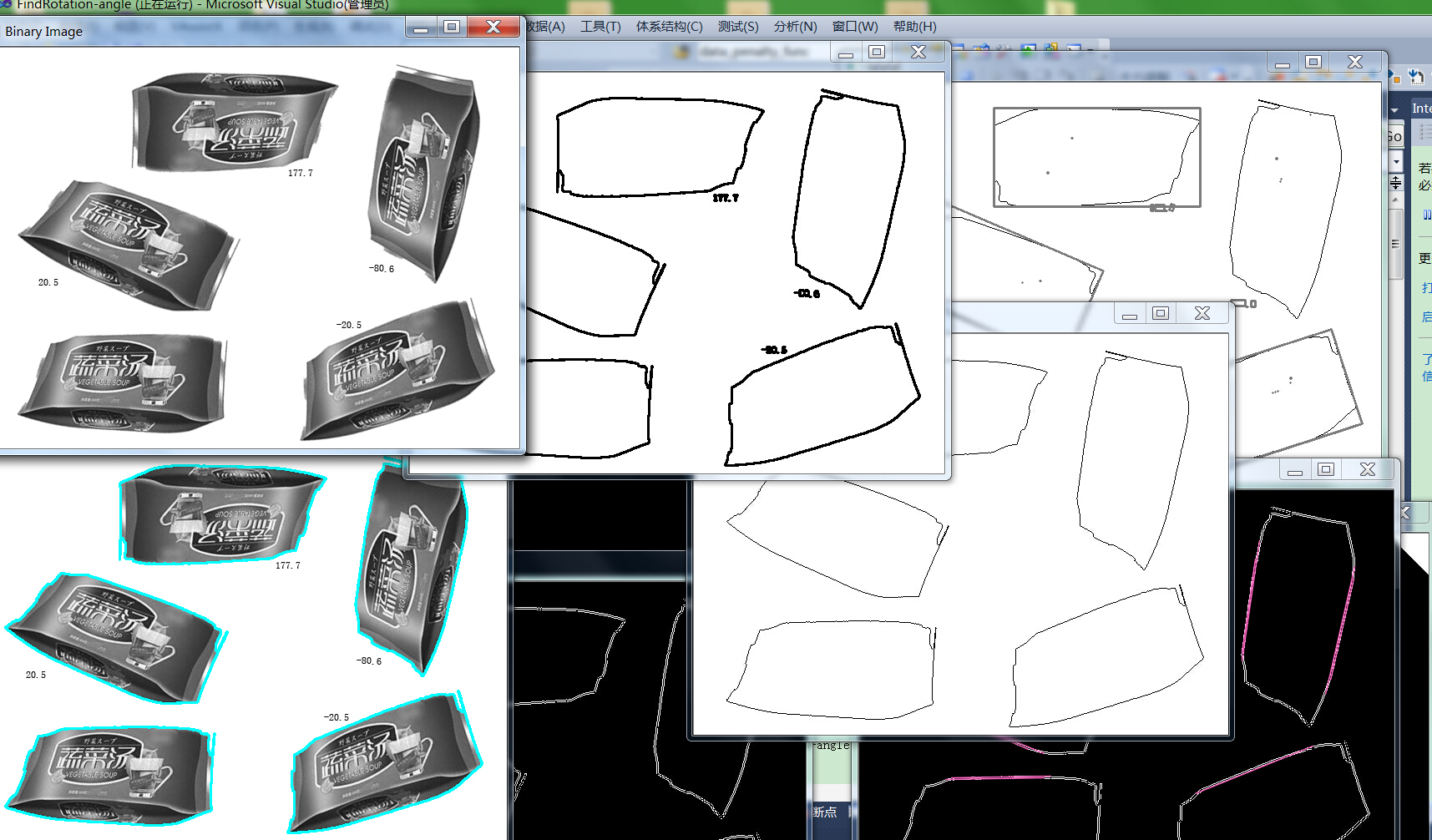这个是原来实现的代码的博客文章：
http://blog.csdn.net/wangyaninglm/article/details/41864251

参考文献：
http://blog.csdn.net/z397164725/article/details/7248096
http://blog.csdn.net/fdl19881/article/details/6730112
http://blog.csdn.net/mine1024/article/details/6044856

展开全文• 在做项目时，很时候可能需要做旋转功能，这个很简单，直接使用Rotate方法即可，如果在加上一个限制旋转角度，可不是简单的判断下角度就可以了。在Unity中，角度在它内部是用四元数表示的，这个我也弄不清楚，所以...
在做项目时，很多时候可能需要做旋转功能，这个很简单，直接使用Rotate方法即可，如果在加上一个限制旋转角度，可不是简单的判断下角度就可以了。在Unity中，角度在它内部是用四元数表示的，这个我也弄不清楚，所以，在面板展示和你实际打印出来的localEulerAngles不一样！！可能你只是想改变x的角度，但是你转着转着可能看到变成x，y，z三个值都变了。

之前在网上看到一个解决方案，说是拿取localEulerAngles之后，对角度做一个变化：

float CheckAngle(float value){
float angle = value - 180;
if (angle > 0){
return angle - 180;
}
return angle + 180;
}

这个虽然可以解决部分问题，但是我这里还是遇到了一个很奇特的问题，有时候突然变成反向的了。

我自己加了一个方法：

float angle = 0;
Vector3 axis = Vector3.left;
rotateXAnim.localRotation.ToAngleAxis(out angle, out axis);

这个可以得到某一个轴的角度，然后在根据axis的方向，就能得到实际的角度

angle = CheckAngle(angle*axis.x);

if (angle > 90){
angle = 90;
rotateXAnim.localEulerAngles = new Vector3(angle, 0, 0);
}else if (angle < -90){
angle = -90;
rotateXAnim.localEulerAngles = new Vector3(angle, 0, 0);
}

结合起来，也能解决大部分问题。

可是，今天又出现一个奇怪问题，就是我前面说的，突然变成反向的角度，而且只有第一次出现，之后旋转都是正常的。

思来想去，最后想到一个曲线解决方案：

我们在初始化物体时，是知道物体的旋转角度的，那么我们就可以存储一个临时的欧拉角度，在需要旋转角度时，直接用这个临时的欧拉角度进行计算，计算得到结果之后，直接先判断角度是否超过我们给定的值，这样就不用经过四元数那一层计算了，那么也就不会出现万向锁和我上面说的问题了。

void MapRotating(Vector3 tempPosition){
Vector3 tempAngle = Vector3.zero;
tempAngle.x = tempPosition.y * 15f / scaleTrans.localScale.x;
tempAngle.y = -tempPosition.x * 15f / scaleTrans.localScale.x;
nowRotate += tempAngle;
nowRotate.x = ClampAngle(nowRotate.x, -90, 90);
SetNowRotValue(nowRotate.x, nowRotate.y, true);
}
static float ClampAngle(float angle, float min, float max) {
if (angle < -360){
angle += 360;
}
if (angle > 360){
angle -= 360;
}
return Mathf.Clamp(angle, min, max);
}

哈哈，想问题真的进入到一个思维死角里面去了，反而忘记后面这个最简单的方式了-
展开全文Unity3D
• 以上就是我的问题，有点，或许是因为这个旋转方法我使用的不对，如果不这样做，有什么方法可以让物体以一定的速度旋转一个角度呢？ 还请大佬指点一下，我将不胜感激。我的wx是： Y2548962922c# c#
• 问题：相机始终围绕着O...条件：abcd这个矩形盒差不多是物体O的顶视图尺寸，A点箭头是相机所在的起点和角度，B点为目标点需要观察的角度。 直接直线移动相机并按照AB夹角的差去移动和扭转相机的话 镜头很容易翻转！
• 例如Chart贴于正前方，分别从Chart的左侧面、上侧面拍摄，或者将摄像机沿着Chart所在平面的法向量进行旋转后拍摄，现在要反求出这三种情况下的旋转角度，用来矫正摄像头与被拍摄物体角度! [Chart图如下]...
• 网上关于物体旋转的方法非常，但大都是单一轴旋转，或指定的角度旋转比如：（Vector3.up 或（0,90,0））； 由于旋转的坑太，会发现将代码套用在我们的需求下后产生一种问题，即在单一轴旋转时其他两个轴的旋转...
• 专家们想了想，利用类似红外线来检测，买了很高级设备，厂家花了很钱，终于把这件事解决了。可是另外一个厂，他们是将电风扇放在流水线前面，利用吹风，把轻的空盒子吹掉，来解决这一问题。  上面的故事对
• ## 物体检测

千次阅读 2018-06-11 21:18:32
同时基本上所有的检测任务都需要在给出物体的bounding box之后，给出物体的类别（也就是给识别物体），所以文章中不区分检测和识别任务。笔者从事计算机视觉中目标检测相关的工作还比较，将自己看到的，学到的，...
• 一个物体在空间中的六个自由度中有三个旋转角度，如下图所示所有坐标系遵循右手规则。我们定义该物体的欧拉角[r p y]表示：绕x轴的为r (roll)即翻滚角，绕y轴的为p (pitch)即俯仰角，绕y轴的为y (yaw)即偏航角。那么...人工智能
• 通过离线收集海量图片和视频样本，人为标定样本后提取特征值，基于特征值与样本标定进行训练进而设计出高效的特征选择分类器。 在实时系统中，使用特征...可同时检测物体，如人脸、人体、车体、车牌、车标、交通
• 看最近物体识别互动桌比较火 本人就自己开发了一套物体识别底层开发包，以Unity插件的形式调用 CSDN好像放不了视频 先看效果！案例视频均为基于此开发包开发的程序，案列视频链接： ...AI 逆向运动学 方法
• 那么问题来了：如果在远处有一个物体，我们需要测量其角度，该如何测量？ 可能吗？ 最简单的例子，假设警察探案，在对面的楼上看到嫌疑所在的屋子的窗子打开了一些，如果警察要确认窗子打开了多少该怎么办？ 大概...APP iOS
• 总结一些物体惯性旋转方式，项目中用到了第二种，也推荐旋转相机，可以用一个单独的相机照射模型。另外，触控屏获取 Input.GetAxis(“Mouse X”) 获取不到，使用 Input.touches.deltaPosition.x 来代替。 物体...
• 如何设置一个物体为另外一个物体的子类 如何动态生成物体 可以参考：unity动态创建游戏物体并放置到一个父类游戏物体下  Unity通过脚本实现给一个物体添加子物体 Unity实例化对象的公共...Unity
• 我的思路是在一个虚拟物体上建立一个参数，将这个参数通过脚本控制场景中所以有circle物体Y轴的旋转，每个circle物体根据自己的序号旋转不同的角度。弄了半天，遇到一些麻烦：max的script似乎只能在每次运行的时候...parameters float
• Unity中控制物体运动控制物体上下左右移动控制物体转向和前后移动       在介绍控制物体运动时，首先需要了解一些基本知识：       1. Input类的常用方法 方法 内容 GetAxis ...unity
• Vuforia sdk 4.0的新功能就是物体识别Object Recognition和物体扫描器 vuforia object scanner，制作一个手表扫描案例简单说一下使用流程。 Vuforia 对象扫描仪使用有四个阶段 设置-应用于设置环境。 扫描-捕捉...unity 技术 Vuforia
• 2-D物体任意角度的反弹 -Twinsen编写 -本人水平有限，疏忽错误在所难免，还请各位数学高手、编程高手不吝赐教 -我的Email-address: popyy@netease.com 第一次我说了一下向量知识的基础内容和一点使用技巧，...float c 算法
• 物体识别的目的就是要找到一个包含可以区分不同目标物体的有效信息的描述。由于要识别的物体是事先知道的，所以目标物体的几何特征可以被直接应用到识别任务中。 　不规则物体的形状匹配是一种有效...
• 使用Python和OpenCV检测图像中的物体并将物体裁剪下来
• ## 物体的形状识别

万次阅读 2014-10-06 10:54:30
物体的形状识别是模式识别中一个基本问题，也是一个重要问题，其广泛应用于图像分析、计算机视觉和目标识别等领域。人类可以很容易地识别物体的形状，但是对于计算机来说，自动识别任意物体的形状却相当困难。...
• ## ARFoundation之路－3D物体识别之一

千次阅读 热门讨论 2019-08-14 14:02:37
3D物体跟踪技术的基础也是图像识别，但比前述图像识别跟踪要复杂得，原因在于现实世界中的物体是三维的，从不同角度看到的物体形状、纹理都不一样，在进行图像对比时需要的数据比2D图像识别要。   在...
• ## 关于准确抓取物体

千次阅读 2015-04-28 16:47:58
在准确抓取物体这件事情上，我花费的时间应该不止两个月了，期间也了解了不同的方法，然后还是抓取物体不准确。最近还是不死心，感觉自己都花费了这么时间了，还怕再花点儿时间嘛。不过，还是打算把最近了解的...
• ## 测量图片中物体大小

千次阅读 多人点赞 2020-05-10 20:35:05
测量图片中物体直径边缘探测旋转图像图像去除黑边测量宽度计算图形旋转角度对图像加工（核心）使用总结 昨天和今天没有学习，有个学弟找我帮忙做个小demo，说要交差应付一下作业。想法是测量一下图片中近似于圆柱的...图像识别
•网络 算法 object 图像处理...